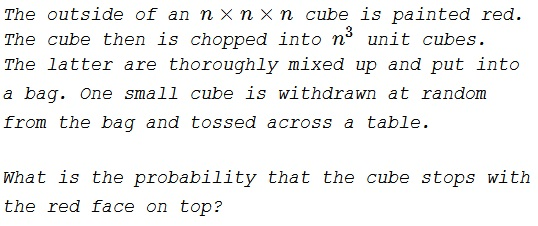# Red Faces of a Cube

### Problem### Solution 1

All faces are equiprobable, making the sought probability equal to $\displaystyle \frac{6n^2}{6n^3}=\frac{1}{n}.$

### Solution 2

For $n=1$, the probability is $1$ as all faces of the cube are painted.

For $n\gt 1$, let $f(k,n)$ be the number of unit cubes that have $k$ faces painted.

$\displaystyle f(k,n)= \begin{cases} (n-2)^3,~\text{for$k=0$} \\ 6(n-2)^2,~\text{for$k=1$} \\ 12(n-2),~\text{for$k=2$} \\ 8,~\text{for$k=3$} \\ 0,~\text{for$4\leq k\leq 6$}. \end{cases}$

Thus, the required probability is

\displaystyle \begin{align} P(n)&=\sum_{k=0}^{6} P(\text{red face up}|\text{cube hask$faces painted})P(\text{cube has$kfaces painted}) \\ &=\sum_{k=0}^{6}\frac{k}{6}\cdot\frac{f(k,n)}{n^3} \\ &=\frac{1}{6n^3}\left[6(n-2)^2+24(n-2)+24\right] \\ &=\frac{1}{n}. \end{align}

Wondering how we get such a nice looking answer. There are a total of $6n^3$ faces out of which $6n^2$ are on the outer surface. Choosing a random cube and a random face is equivalent to choosing a face randomly from the $6n^3$ possibilities.

### Acknowledgment

The problem 948 from the Pi Mu Epsilon Journal (Fall 1999, p 56). It was included in Ross Honsberger's Mathematical Delights (MAA, 2004, p 78).

Solution 2 is by Amit Itagi.